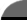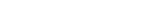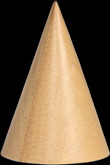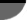Facts By Category:» Physics  » Astronomy  » Chemistry  » Biology  » Mathematics  » Geology  » Engineering  » Medicine  » Science   by keyword search:       ScienceIQ Team:»Writers & EditorsxUmp.com
Science Supplies,

Physics & Astronomy
Online
How To Calculate The Area Of A Right ConeThe cone is another three-dimensional shape based on the circle. You could think of it as the cross between a circle and a right triangle. Its properties will have features of both shapes, and this makes it easier to understand. One way to visualize a right cone is to think of it as a cylinder that tapers continuously to a point The area of a normal cylinder is obtained by summing the area of the body and of the two circles that make up the ends. A right cone, however, only has one circular end so the expression for its area will include only one circle. This leaves only the area of the body of the cone to determine. Any right cone can be thought of in two other ways. A right cone can be viewed as a stack of circles in which each circle is smaller than the one before it. The last one is nothing more than a point on the central axis that passes through each circle for the length of the stack. Now imagine that the cone has been cut in half along this central line.

Looking directly toward the cut surface, one would see a triangle with two equal angles at the base (an isosceles triangle). But looking directly along the cut surface, one would see a right triangle. This right triangle is the key to the area of a right cone. Imagine that right triangle being turned all the way around a complete circle along its vertical side. The result is the right cone that you started with. Clearly the area of the body of that cone has been described by the hypotenuse of the triangle as it traveled around in a circle, and the area of the base of the cone has been described by the base of the triangle as it traveled around. The total surface area of the right cone is therefore the sum of these two areas. The area of the base is given by the general formula for the area of a circle. The area of the body is given by the length of the hypotenuse of the right triangle multiplied by p and by the radius of the circle.

The total area of the right cone is then given by adding these two areas together to get the general equation A = prh + pr2. As an example of how to use this equation, imagine that you want to make a cloth tent to use as a cabana or changing room at the beach. The main body of the cabana will be made from a large piece of cloth that you already have, but you need to find out how much more material you need to buy in order to make the peak and floor of the cabana. You know that the cabana will be 4 feet wide and 5 feet high. The peak of the cabana should be 6 feet high, so the vertical height of the cone will be 1 foot, and the radius will be 2 feet. The hypotenuse of the angled side of the peak is calculated by h2 = r2 + l2 = 4 + 1 = 5 h = 5. This means that you will have to have at least A = prh + pr2 = (3.14 X 2 X 5) + (3.14 X 4) = 14.13 + 12.56 = 26.69 square feet of extra material to complete the cabana.

476Richard M. J. Renneboog, MS Richard M. J. Renneboog is an independent private technical consultant and writer in both chemical and computer applications. Endeavors have included preparation of scripts for instructional and promotional video, corporate website design, curriculum development for training in advanced composites technology, and development.Geometry the Easy Way (Geometry the Easy Way, 3rd Ed)by Lawrence S. Leff
 Home | Privacy Policy | Cookie PolicyCopyright © 2002-2019 ScienceIQ.com - All Rights Reserved